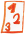Custom math worksheets at your fingertips# Details for problem "Area of triangle draw triangle and height and measure"

Quickname: 4289

## Summary

A triangle has to be drawn with given dimensions. Draw a height of choice, measure side and height and calculate area.

## Example## Description

Some dimensions of a triangle are given that unambiguously specify the triangle. The task is to draw this driangle, then determine its area by measuring one of the sides and the corrsponding height.

The dimensions given can be chosen to be either

- the lengths of the three sides

- the site of two angles and the length of the common side

- the length of two sides and the size of the angle between these sides

- or a random selection of the three possibilities above.

Now the following steps have to be undertaken:

- draw any height

- measure the length of the height ha and the corresponding side a

- use the formula T = a * ha / 2 to calculate the area of the triangle.

The number of problems is selectable.

Download free worksheets for this math problem here. The worksheet contains the problems only, the solutions sheet includes the answers. Just click on the respective link.

•Worksheet 1Solution sheet with answers
•Worksheet 2Solution sheet with answers
•Worksheet 3Solution sheet with answers

If you can not see the solution sheets for download, they may be filtered out by an ad blocker that you may have installed. If this is the case, please allow ads for this page and reload the page. The solution sheets will then reappear.

These worksheets contain math problems that have to be printed with the correct aspect ratio. When printing, please take care to switch all automatic adjustments, scaling of page, auto adjust, etc, off.

• Do the sample worksheets do not really fit?
• Do you need more math worksheets, with a different level of difficulty?
• Would you like to combine different problems on a worksheet and adjust them to your needs?
• As a teacher, you can put together your own worksheets using the automatically generated math problems provided.
With a free initial credit, you can start creating your own math worksheets in a few minutes.

It does not cost anything to try! Register here, to create custom worksheets now!

## Customization options for this problem

Parameter
Possible values
Number of problems
1, 2
Given
sides, angle,side,angle, side,angle,side, sas or asa, sss or sas, asa or sas, sss, sas or asaDeutsche Version dieser Aufgabe
These informational pages with samples describe math problems that can be combined on custom math worksheets with solutions for home and school use.
Deutsche Seiten
×